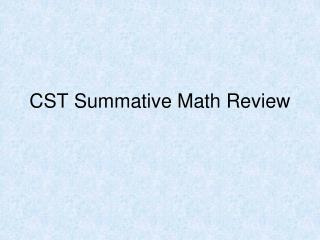DownloadDownload PresentationCST Summative Math Review

CST Summative Math Review

Download PresentationCST Summative Math Review

- - - - - - - - - - - - - - - - - - - - - - - - - - - E N D - - - - - - - - - - - - - - - - - - - - - - - - - - -
Presentation Transcript

1. CST Summative Math Review

2. Algebra 1 Standards • 18 questions – 28% of test • Test includes questions from the following categories: • Number Properties, Operations, & Linear Eqns • Graphing & Systems of Linear Eqns • Quadratics & Polynomials • Functions & Rational Expressions

3. Algebra II Standards • 23 questions – 35% of the test • Categories include: • Polynomials & Rational Expressions • Quadratics, Conics & Complex Numbers • Exponents & Logarithms • Series, Combinatorics, & Probability & Statistics

4. Geometry Standards • 19 questions – 29% of the test • Questions fall into the following categories • Logic & geometric proofs • Volume & area formulas • Angle relationships, constructions, & lines • trigonometry

5. Probability & Statistics Standards • 5 questions – 8% of the test • Test includes questions from the following: • Probability of independent events • Conditional probability • Variance & standard deviation

6. 3. Which equation is equivalent to 5x −2( 7 x +1) =14x? A −9x-2 =14x B −9x+1=14x C −9x+2 =14x D 12x−1=14x 4. Which equation is equivalent to 33 − 5x = 6− 3(1−3x)? A -7x = 30 B 7x = -30 C 7x = 15 D -7x = -15

7. 5. “Two lines in a plane always intersect in exactly one point.” • Which of the following best describes a counterexample to the assertion above? • Coplanar lines • Parallel lines • Perpendicular lines • Intersecting lines • 6. Which figure can serve as a counterexample to the conjecture below? • If one pair of opposite sides of a quadrilateral is parallel, then the quadrilateral is a parallelogram. • Rectangle • Rhomus • Square • trapezoid

8. What is the probability of tossing a six-sided die twice and getting a sum of 5? 1/18 2/9 1/9 1/36 What is the probability of drawing two yellow marbles (without replacement) from a bag containing 3 green, 6 yellow, and 5 red marbles? 15/91 18/91 1/3 3/4

9. 9. Which equation is equivalent to 3[7x − 4 (x−3) ]+1=16 ? A 9x−2=16 B 9x+37 =16 C 17x−2=16 D 17x+13 =16 10. The total cost (c) in dollars of renting a sailboat for n days is given by the equation c =120 +60n. If the total cost was \$360, for how many days was the sailboat rented? A 2 B 4 C 6 D 8

10. 11. Given: TRAP is an isosceles trapezoid with diagonals RP and TA. Which of the following must be true? • RP ┴ TA • RP ║ TA • RP TA • RP bisects TA • 12. Which triangles must be similar? • Two obtuse triangles • Two scalene triangles with congruent bases • Two right triangles • Two isosceles triangles with congruent vertex angles

11. What is the probability of selecting a face card or a 2 from a standard deck of playing cards? 3/13 12/13 11/13 4/13 If the probability of an event happening is P(E)=0.72, what is the probability that the event will not happen? 0.72 0.14 0.28 1

12. t 1 l 2 m 18. In the accompanying diagram, parallel lines l and m are cut by transversal t.Which statement about angles 1 and 2 must be true? • 1 and 2 are congruent • 1 is the complement of 2 • 1 is the supplement of 2 • 1 and 2 are right angles

13. N O 3a-2b M P 4a+b 19. What values of a and b make quadrilateral MNOP a parallelogram? 21 13

14. 20. Which point lies on the line defined by 3x+6y=2? A (0, 2) B (0, 6) C (1, -1/6) D (1, -1/3) 21. What is the equation of the line that has a slope of 4 and passes through the point(3, -10)? A y=4x-22 B y=4x+22 C y=4x-43 D y=4x+43